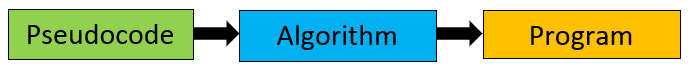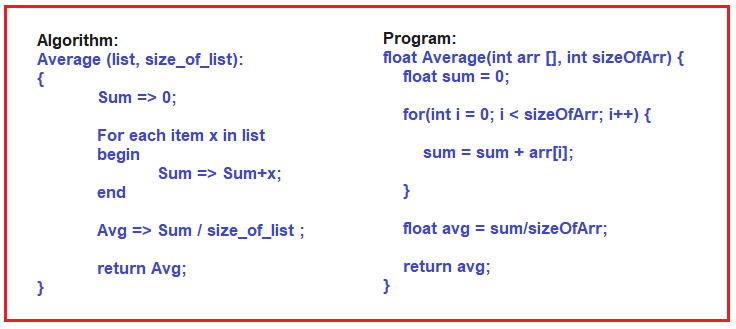# Algorithms, Pseudocode, and Program

## What are Algorithm, Pseudocode, and Programs

In this article, I will give you a brief introduction to What are Algorithms, Pseudocode, and Programs. At the end of this article, you will understand what are Algorithms, Pseudocode, and programs.

Algorithm: An algorithm is a step-by-step procedure for solving a computational problem. It is a process or set of rules to be followed in calculations or other problem-solving operations.

Program: Program is a step-by-step machine instruction used for solving any problem or computational task.##### Difference between Algorithm and Program

Programs have been written recently but Algorithms have appeared for centuries. As a common practice, mathematicians or scientists have been devising procedures for solving computational problems. Those working on problems were giving solutions in form of step-by-step procedures known as algorithms. Now we want that same procedure must be followed by machines so we are writing programs.

An algorithm basically means how to solve a problem. First, we need to learn a few analytical or problem-solving skills to write an algorithm.

###### Example:
• Let’s consider a chef who knows how to prepare a dish then he/she can easily prepare the recipe of that dish.
• Let’s consider a chemist who is well versed with different chemical reactions then he/she can easily prepare a chemical formula applying those reactions.

Once an algorithm is prepared, we need to convert it into a Program so that the computer can execute it and perform the computational task.

Any programming language can be used to write a program but it must strictly follow the syntax of that programming language.

##### Pseudo Code

Pseudocode is an artificial and informal language that helps programmers in developing algorithms. It is basically a “text-based” detail (algorithmic) design tool.

##### Algorithm Example:

So here I have an example algorithm as well as a C++ program that is not a complete program is just a function.##### Let us Understand the Algorithm.

The Algorithm is for finding the average of the list of elements. That is, we have a collection of elements and we want to find out the average. First, we assign 0 to Sum. Then for each element x in the list, we begin sum assigned sum+ x i.e. adding each value of x into the sum variable. Then after that, the average is assigned sum by the number of elements, and then, return the average. So, if you read the above algorithm, you can understand how to find the average of a list of elements. Add all of them and divide by the number of elements. That’s it. This is how we write our algorithm using pseudocode.

##### Let us Understand the Program.

Now the same thing for finding the average list of elements, we have written the program using C++ language. It’s a function, it’s not a complete program, just a function inside a program. If we don’t use a semicolon to end the statement, it’s an error, and instead of assignment if we write less than or a hyphen symbol, then also it is an error. So, if you want to store the value then you must use an equal symbol and that is called an assignment.

So, it means you should follow the proper syntax of a language. Because this is not for you. You are writing the program for the compiler to understand and convert it into machine code. You will write a C++ program and that gets converted into machine code or machine language. So, you are actually talking to the compiler. You should talk in such a way that you can easily understand.

If the compiler is not understanding your program, then the compiler cannot convert your program into machine code. So, you should follow the syntax perfectly. That is the reason you have to put some little extra effort into learning programming.

That’s all about the comparisons of Algorithms, Pseudocode, and Programs.

In the next article, I am going to discuss What is Flowchart. Here, in this article, I try to explain what are Algorithms, Pseudocodes, and Programs and the differences between them and I hope you enjoy this algorithm, Pseudocodes, and Programs article.